# Three-Color Cellular Automaton Rules that Double Their Input

A list of rules for k=3 cellular automata that eventually double a block of gray input cells

Originator: Stephen Wolfram

A collection of these rules originally appears on page 833 of A New Kind of Science (Wolfram, 2002).

The rules presented are the 199 minimum representatives of equivalence classes of rules. (The total raw number of rules is 4277.)

## Examples

### Basic Examples

Retrieve the resource:

 In:=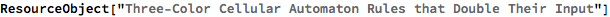Out=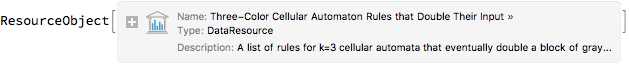Retrieve a sample of the default content:

 In:=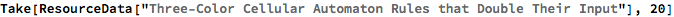Out=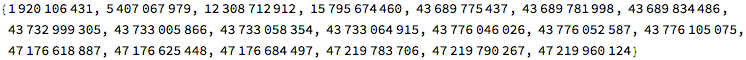Pick 10 random examples of these rules:

 In:=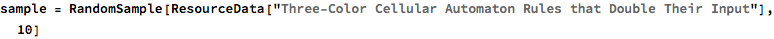Out=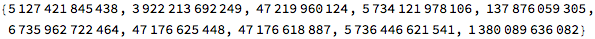See how they act on inputs of length 10:

 In:=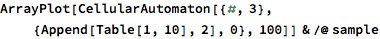Out=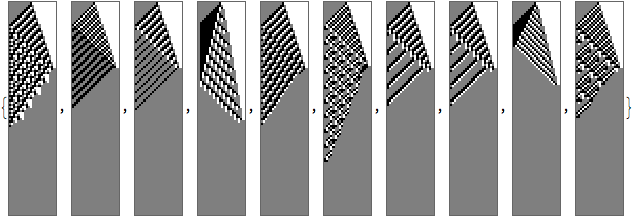See that this holds when input length and evaluation steps are doubled:

 In:=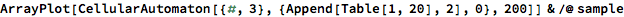Out=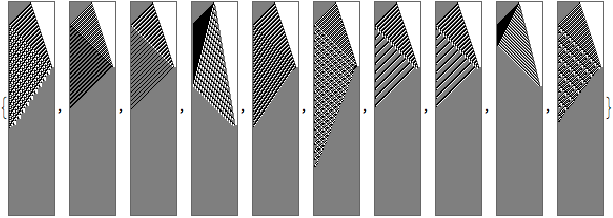Find how many steps each rule takes to “settle down” from an initial conditions with 20 1s:

 In:=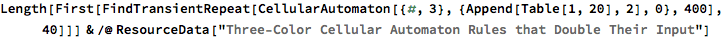Out=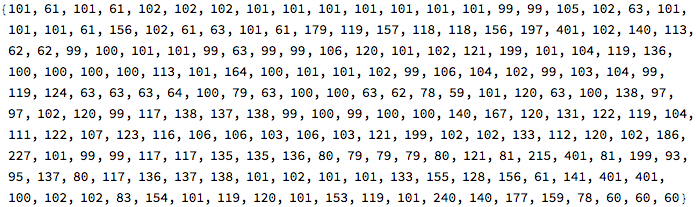Find the 5 rules with the longest transients:

 In:=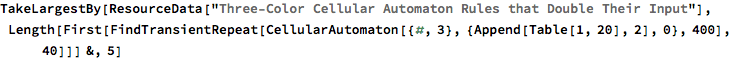Out=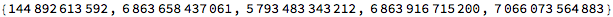Show the behavior for these rules:

 In:=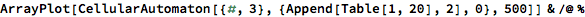Out=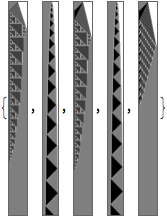Wolfram Research, "Three-Color Cellular Automaton Rules that Double Their Input" from the Wolfram Data Repository (2017) https://doi.org/10.24097/wolfram.07085.data